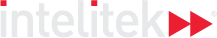### Course Overview

Kinematics covers the basics of motion. We begin with the units used for speed, time and displacement, and graphs of motion at a constant velocity. Next is acceleration and the equations for motion, followed by the motion of falling objects, gravitational acceleration, and projectile motion. The final lesson covers circular motion, period and frequency.

HOURS OF INSTRUCTION: 15

### Course Outline

• Basics of Motion
• Uniformly Accelerated Motion
• Free-fall Motion
• Projectile Motion
• Circular Motion